# High School Math : Other Polygons

## Example Questions

1 3 Next →

### Example Question #7 : How To Find An Angle In A Polygon

What is the measure of one interior angle of a regular twenty-three-sided polygon (nearest tenth of a degree)?

Possible Answers: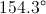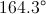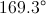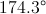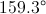Correct answer:Explanation:

The measure of each interior angle of a regular polygon withsides is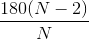. We can substitute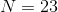to obtain the angle measure: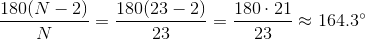### Example Question #8 : How To Find An Angle In A Polygon

A regular polygon has interior angles which measure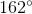each. How many sides does the polygon have?

Possible Answers:Correct answer:Explanation:

The easiest way to answer this is to note that, since an interior angle and an exterior angle form a linear pair - and thus, a supplementary pair - each exterior angle would have measure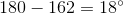. Since 360 divided by the number of sides of a regular polygon is equal to the measure of one of its exterior angles, we are seekingsuch that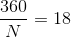Solve for: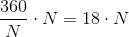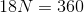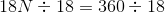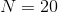The polygon has 20 sides.

### Example Question #1 : How To Find The Length Of A Side Of A Polygon

If the area of a regular octagon is 160 and the apothem is 8, what is the side length?

Possible Answers: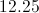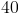Correct answer:Explanation:

To find the side length from the area of an octagon and the apothem we must use the area of a polygon which is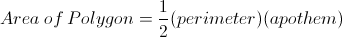First plug in our numbers for area and the apothem to get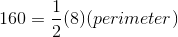Then multiply to get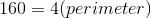Then divide both sides by 4 to get the perimeter of the figure.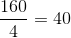When we have the perimeter of a regular polygon, to find the side length we must divide by the number of sides of the polygon, in this case 8.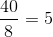After dividing we find the side length is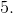1 3 Next →

### All High School Math Resources